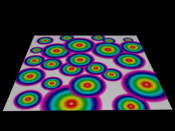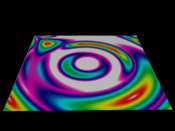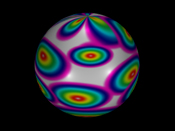"Swirling" Texture Maps

In this challenge we were asked to create a shader that would take the information from a texture map and then "swirl" or "stretch" the map across the s and t values.

To start with this shader I needed to do some research on how to move the values. We were told that polar coordinates might help in this, so that is where I started. Finding information on the web I was able to find this:

This shows how a polar coordinate is calculated from the origin.

O = the origin ( x = 0, y = 0)

r = the distance of the point from the origin

P = the point coordinates (x, y)

Theta = is the polar angle, angle of r from x in a counter-clockwise direction

So, with these defined we just have to convert the math into something that the shader can understand.

First off, x = s. y = t. Now to get the other values we need to use some basic mathmatics.

r = sqrt((x * x) + (y * y)) or sqrt((s * s) + (t * t))

Theta = arctan(y/x) or atan(t/s)

So with this math in place I was able to get a shader that allows for the texture to be morphed around the origin, which is the top left corner of the polygon. Eventhough it was interesting to see, it was not what we wanted, we want the texture to morph around the center of the polygon and not the origin. I then offset the s and t values within the shader by 0.5 which centered the origin in the middle of the polygon. I set this as a parameter within the shader so that the artist would be able to move the "origin" to wherever they want. So with the values centered I was able to get an idea of what the shader would look like. Knowing that what like is not necessarily what every one likes I put in another parameter that allows the artist to "tweek" the theta value by multiplying by whatever number the artist puts in. To allow for more freedom to the artist, I set parameters for both the s and t offset values and s and t theta values. The images below show the before and after the shader is applied to the polygon.click to enlargeclick to enlarge click for code

As you can see, it does morph the texture. I decided that I would like to see how it would look applied to a sphere.click to enlargeclick to enlarge

I would like to use this on a picture texture to see exactly how the morphing works. But I am satified that this shader seems to be holding up with the image that I am using here. A few problems that might arise is that when you start increasing the theta values the image starts to tile across the polygon. Not sure why this happens, but it might be because of the simplicity of the math that I have concluded to above. There might be a better more elegant way to arrive at the same shader that I have created here. It is definately something that could be explored further.